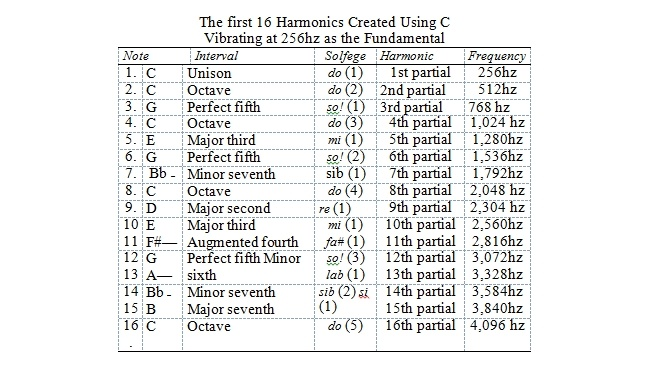Science of Harmonics by Jonathan Goldman

What Is Sound?

The universe is alive with sound and within all sounds are harmonics. Harmonics, also known as ‘overtones’, are a phenomenon of sound that occurs whenever sound is created. Normally we perceive what seem like single tones when we hear a note struck on a musical instrument like a violin or a piano. However, nearly all tones that are produced by musical instruments, our voices or other sound sources are in reality not pure tones, but mixtures of pure tone frequencies called ‘partials’. The lowest such frequency is called the fundamental. All partials higher in frequency than the fundamental are referred to as overtones.

Before we begin our exploration of harmonics as a phenomenon of sound, let us first examine sound. Sound is a vibrational energy which takes the form of waves. These waves are scientifically measured in units called hertz (‘hz’) which measure the cycles per second which this energy creates. This rate is objectively known as the ‘frequency’. It is subjectively experienced as ‘pitch’.

Frequency A string that vibrates back and forth one hundred times a second will create a sound that can be measured at 100hz. This would be its frequency. One that vibrates one thousand times a second would be measured at 1000 hz. Overtones are mathematically related to each other. Remember the example of the string which vibrated at 256 and created the note C? As that string vibrates 256 times, other sound waves are also being created that are vibrating as geometric multiples of 256 hz. The first of these overtones to sound is vibrating twice as fast as that fundamental, at a ratio of two to one (2:1), at 512 cycles a second. This creates a note which is referred to as the interval of an octave of the fundamental and is also called a C.

The First 16 Harmonics The table below shows the first 16 harmonics created using C vibrating at 256 hz as the fundamental. It also shows the Solfège name of the overtone being created, its frequency and the interval created. The first column represents the note name given to the harmonic. The second column shows the interval within the octave created by the harmonic. The third column is the Solfège name given for the harmonic (the parenthesis represents the number of times they appear). The fourth column represents the partial names for the harmonic. The fifth column shows the frequency of the harmonic.